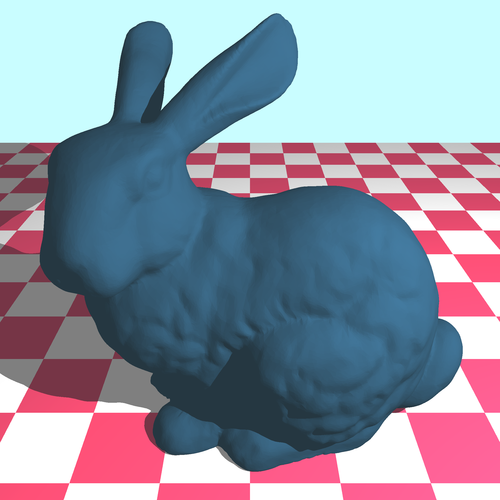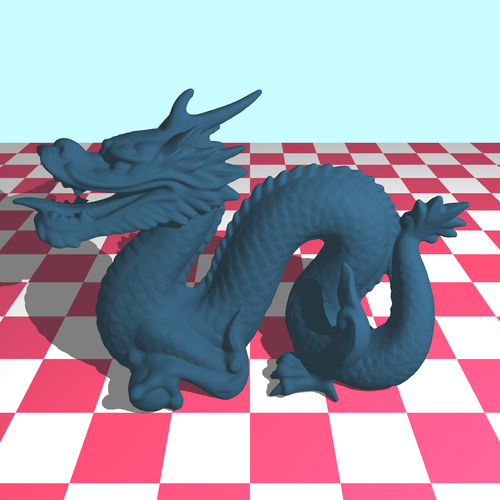18 May 2019

After completing our efficient queryable structure (and a lot of debugging!), we wanted to test our program on more complex scenes. One of our goals was to load arbitrary models from a file. A common file format that very simply describes a 3D model STL. The model does not contain any color information and the description is merely just a triangle mesh: precisely what we can process.

The informations are encoded in binary in the following way:

Data Type Comment
char Textual header
uint32 The number of triangles
Triangle[] The triangles

The Triangle structure is defined as follows:

Data Type Comment
Vector The normal vector $\vec{n}$
Vector The vertices $v_0$, $v_1$ and $v_2$
uint16 A checksum

And finally Vector is defined as:

Data Type Comment
float32 The $x$, $y$ and $z$ coordinates

Here are the results of some of our tests with some STL models that are in the public domain:The Utah teapot on a checkerboard pattern rendered with a focus blur effect. This scene contains around 10,000 triangles in total. The rendering took 15 minutes with all optimizations enabled and a sampling rate of 400 rays per pixel.The Stanford bunny, around 87,000 triangles; rendering with a $4 \times 4$ supersample (antialiasing) took 90 seconds.The Stanford dragon, around 300,000 triangles; rendering with the same parameters as the bunny took 120 seconds.

As you can see the complexity of the scene now barely affects the rendering time, which is an excellent news.